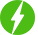# Numerical Methods with Python: Bridging Theory and Practice

1

Numerical Methods with Python: Bridging Theory and Practice, Numerical Methods and Analysis for Engineers and Scientists.

## Course Description

Explore the fascinating world of numerical methods and unlock the power of Python programming language for solving complex mathematical and physical problems. In this comprehensive course, you will delve into the essential theoretical foundations of numerical analysis while gaining hands-on experience with practical implementations using Python.

From root-finding, interpolation and numerical integration to solving differential equations and optimization, this course equips you with the necessary mathematical knowledge and programming skills to tackle a wide range of real-world challenges. You’ll learn to apply numerical algorithms, understand their strengths and limitations, and analyze their accuracy through rigorous error analysis.

Designed for both aspiring mathematicians and Python enthusiasts, this course strikes a perfect balance between theory and application. Through engaging lectures, interactive coding exercises, and real-world projects, you’ll build a strong understanding of numerical methods’ underlying principles and learn to implement them effectively with Python libraries like NumPy and SciPy.

Whether you aim to optimize engineering designs, simulate physical phenomena, analyze financial data, or delve into data science, “Numerical Methods with Python” empowers you to confidently approach diverse problems with numerical precision. Join us on this exciting journey, and elevate your problem-solving capabilities to new heights with the synergy of mathematics and Python programming

We will be happy to hear your thoughtsRegister New Account
• Total (0)
0